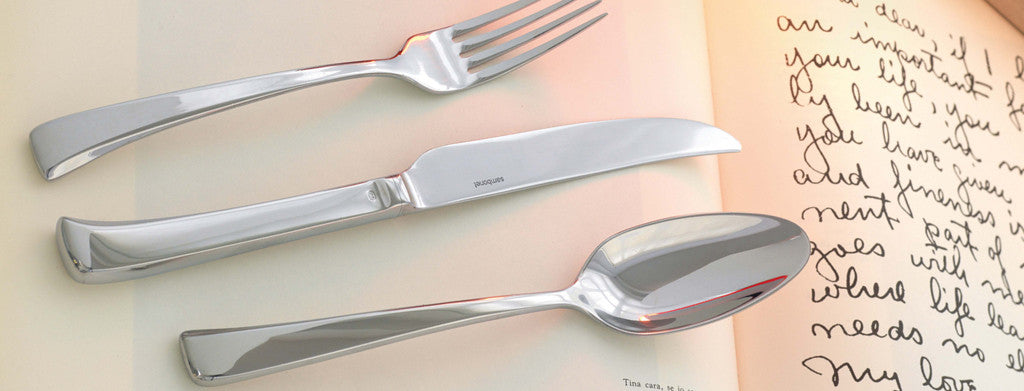Shopping Cart

### Refine

View all

#### Price# Cutlery£3.70 each (ex VAT)£2.94 each (ex VAT)£7.03 each (ex VAT)£9.07 each (ex VAT)£5.49 each (ex VAT)£2.56 each (ex VAT)£7.79 each (ex VAT)£0.27 each (ex VAT)£0.48 each (ex VAT)£0.77 each (ex VAT)£2.79 each (ex VAT)£3.04 each (ex VAT)£2.80 each (ex VAT)£6.68 each (ex VAT)£6.68 each (ex VAT)£3.10 each (ex VAT)£21.34 each (ex VAT)£10.03 each (ex VAT)£5.72 each (ex VAT)£19.09 each (ex VAT)£5.72 each (ex VAT)£4.54 each (ex VAT)£8.95 each (ex VAT)£6.45 each (ex VAT)£9.25 each (ex VAT)£7.99 each (ex VAT)£6.45 each (ex VAT)£21.34 each (ex VAT)£5.72 each (ex VAT)£3.29 each (ex VAT)£6.80 each (ex VAT)£4.29 each (ex VAT)£11.21 each (ex VAT)£21.34 each (ex VAT)£6.93 each (ex VAT)£5.01 each (ex VAT)£7.79 each (ex VAT)£7.79 each (ex VAT)£31.59 each (ex VAT)£0.30 each (ex VAT)£0.27 each (ex VAT)£0.91 each (ex VAT)£0.48 each (ex VAT)£0.47 each (ex VAT)£0.85 each (ex VAT)£0.47 each (ex VAT)£1.49 each (ex VAT)£0.67 each (ex VAT)